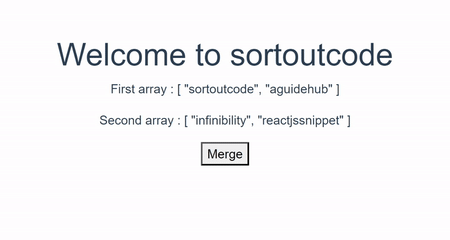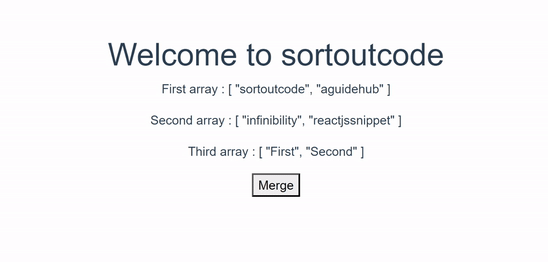# How to merge the array in VueJS?

Hi Friends 👋,

Welcome To SortoutCode! ❤️

To merge the arrays in VueJS. We are going to use `concat()` method for javascript, `\$.merge()` method for Jquery and javascript spread operator `(...)`.

Today, I am going to show you How will you merge two arrays in VueJS?

Table of Content

• Setup the Vue (Optional)
• Create FirstComponent.vue and import it into App.js
• Merge array using Javascript
• Merge array using Jquery
• Merge array using the spread operator
• Merge multiple arrays useing the spread operator

This article will guide you to How to combine two arrays in vueJS.

## Setup the Vue.js

First, we have to install the Vue project, I had installed the vueJS in my system. If you haven’t installed or have any problem with installation you can follow this article, which will show you step by step process of installation.

How to Install the VueJS project?

## Create FirstComponent.vue and import it into App.js

Create the new component in the `src/components/` components folder. the component name is `FirstComponent.vue` and imported into `App.js` file:

`App.js`

``````<template>
<div id="app">
<FirstComponent />
</div>
</template>

<script>
import FirstComponent from "./components/FirstComponent.vue";
export default {
name: "App",
components: {
FirstComponent,
},
};
</script>``````

## Merge array using Javascript

To combine or merge the two arrays using the javascript. We are going to use the `contact ()` method. This `concat()` method will return the final array.

### Short solution

We are going to take the first array `arr1` and the second array that is `arr2`.The final array will contain the merged array value that is `finalarr`.

``````// first array
let arr1 = ["sortoutcode", "aguidehub"];

// second array
let arr2 = ["infinibility", "reactjssnippet"];

// final array
let finalarr = [];

finalarr = arr1.concat(arr2) //  [ "sortoutcode", "aguidehub", "infinibility", "reactjssnippet" ]``````

### Short example

Let’s take the short example of merging two arrays using javascript.

`FirstComponent.vue`

``````<template>
<div>
<h1>Welcome to sortoutcode</h1>
<div>
<p>First array : {{ arr1 }}</p>
<p>Second array : {{ arr2 }}</p>
<p v-if="finalarr.length != 0">Final array : {{ finalarr }}</p>
</div>
</div>
</template>
<script>
import \$ from "jquery";
export default {
name: "FirstComponent",
data() {
return {
arr1: ["sortoutcode", "aguidehub"],
arr2: ["infinibility", "reactjssnippet"],
finalarr: [],
};
},
methods: {
this.finalarr = this.arr1.concat(this.arr2);
},
},
};
</script>``````

## Output

For now, let’s check the output.## Merge array using Jquery

To use Jquery’s all functionality we are going to install the `Jquery` npm package.

### Installation of Jquery

To install jquery in VueJS.we are going to use this command:

``    npm i jquery``

### Short solution

We are going to take the first array `arr1` and the second array that is `arr2`. The final array will contain the merged array value that is `finalarr`.

``````// first array
let arr1 = ["sortoutcode", "aguidehub"];

// second array
let arr2 = ["infinibility", "reactjssnippet"];

// final array
let finalarr = [];

finalarr = \$.merge(arr1, arr2) //  [ "sortoutcode", "aguidehub", "infinibility", "reactjssnippet" ]``````

### Short example

To merge the two arrays using the Jquery. We are going to `\$.merge()` method. This method together merges the content of two arrays into the first array. This method will return the merged array. To use the `Jquery` we have to import the `jquery` package. Let’s take a short example for better understanding. Let’s see the code:

`FirstComponent.vue`

``````<template>
<div>
<h1>Welcome to sortoutcode</h1>
<div>
<p>First array : {{ arr1 }}</p>
<p>Second array : {{ arr2 }}</p>
<p v-if="finalarr.length != 0">Final array : {{ finalarr }}</p>
</div>
</div>
</template>
<script>
import \$ from "jquery";
export default {
name: "FirstComponent",
data() {
return {
arr1: ["sortoutcode", "aguidehub"],
arr2: ["infinibility", "reactjssnippet"],
finalarr: [],
};
},
methods: {
this.finalarr = \$.merge(this.arr1, this.arr2);
},
},
};
</script>``````

## Output

For now, let’s check the output.## Merge array using the spread operator

To combine the two arrays using the javascript spread operator `(...)`. It allows us to quickly copy all or part of an existing array or object into another array or object.

### Short solution

We are going to take the first array `arr1` and the second array that is `arr2`. The final array will content the merged array value that is `finalarr`. And to merge the two arrays we are using the spread operator `[...arr1,...arr2]`.

``````// first array
let arr1 = ["sortoutcode", "aguidehub"];

// second array
let arr2 = ["infinibility", "reactjssnippet"];

// final array
let finalarr = [];

finalarr = [...arr1, ...arr2] //  [ "sortoutcode", "aguidehub", "infinibility", "reactjssnippet" ]``````

### Short Example

Let’s take the short example of merging two arrays using a javascript spread operator.

``````<template>
<div>
<h1>Welcome to sortoutcode</h1>
<div>
<p>First array : {{ arr1 }}</p>
<p>Second array : {{ arr2 }}</p>
<p v-if="finalarr.length != 0">Final array : {{ finalarr }}</p>
</div>
</div>
</template>
<script>
import \$ from "jquery";
export default {
name: "FirstComponent",
data() {
return {
arr1: ["sortoutcode", "aguidehub"],
arr2: ["infinibility", "reactjssnippet"],
finalarr: [],
};
},
methods: {
this.finalarr = [...this.arr1, ...this.arr2];
},
},
};
</script>``````

## Output

For now, let’s check the output.## Merge multiple array useing the spread operator

To merge the multiple arrays, we are using the javascript spread operator `[...array1,...array2,...array3,...arrayn]`.

### Short solution

To merge the multiple arrays that is `arr1,arr2,arr3`. We going to merge all arrays using the spread operator and create a single array that is `finalarr`.

``````// first array
let arr1 = ["sortoutcode", "aguidehub"];

// second array
let arr2 = ["infinibility", "reactjssnippet"];

// third array
let arr3 = ["First", "Second"];

// final array
let finalarr = [];

finalarr = [...arr1, ...arr2, ...arr3] //  [ "sortoutcode", "aguidehub", "infinibility", "reactjssnippet","First","Second"]``````

### Short example

Let’s take a short example and understand how it works:

`FirstComponent.vue`

``````<template>
<div>
<h1>Welcome to sortoutcode</h1>
<div>
<p>First array : {{ arr1 }}</p>
<p>Second array : {{ arr2 }}</p>
<p>Third array : {{ arr3 }}</p>
<p v-if="finalarr.length != 0">Final array : {{ finalarr }}</p>
</div>
</div>
</template>
<script>
import \$ from "jquery";
export default {
name: "FirstComponent",
data() {
return {
arr1: ["sortoutcode", "aguidehub"],
arr2: ["infinibility", "reactjssnippet"],
arr3: ["First", "Second"],
finalarr: [],
};
},
methods: {